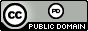시간 제한메모리 제한제출정답맞힌 사람정답 비율
2 초 512 MB31232071.429%

## 문제

Today you are doing your calculus homework, and you are tasked with finding a Lipschitz constant for a function f(x), which is defined for N integer numbers x and produces real values. Formally, the Lipschitz constant for a function f is the smallest real number L such that for any x and y with f(x) and f(y) defined we have:

|f(x) − f(y)| ≤ L · |x − y|.

## 입력

The first line contains N – the number of points for which f is defined. The next N lines each contain an integer x and a real number z, which mean that f(x) = z. Input satisfies the following constraints:

• 2 ≤ N ≤ 200 000.
• All x and z are in the range −109 ≤ x, z ≤ 109.
• All x in the input are distinct.

## 출력

Print one number – the Lipschitz constant. The result will be considered correct if it is within an absolute error of 10−4 from the jury’s answer.

## 예제 입력 1

3
1 1
2 2
3 4


## 예제 출력 1

2


## 예제 입력 2

2
1 4
2 2


## 예제 출력 2

2


## 예제 입력 3

4
-10 6.342
-7 3
46 18.1
2 -34


## 예제 출력 3

4.111111111


## 출처• 문제를 만든 사람: Volodymyr Lyubinets